# Topological Group

(redirected from Closed subgroup)
Also found in: Dictionary.
The following article is from The Great Soviet Encyclopedia (1979). It might be outdated or ideologically biased.

## Topological Group

a mathematical concept arising, like the concept of an ordinary group, in the study of transformations. Suppose M is a set of elements x of a certain kind, for example, numbers, points in space, or functions. We say that there exists a transformation f of M if to every element x in M there corresponds a definite element

(1) yf (x)

in M. It is assumed that for every y there exists a unique x satisfying equation (1). Thus, equation (1) is solvable for x:

x═ f-1 (y)

f-1 is also a transformation of M. The transformation f-1 is called the inverse of f A transformation e, carrying every element x into itself, e (x)x, is called the identity transformation. The successive application of two transformations f and g yields a new transformation k:

k (x)f[g (x)]

The transformation k is called the product of f and g:

kfg

Multiplication of a transformation f by the identity transformation e does not change f:

(2) feeff

The product of a transformation f and its inverse f-1 yields the identity transformation

(3) ff-1f-1fe

Any three transformations obey the associative law:

(4) (fg) hf (gh)

The set of all transformations of a set M is a group. A subset of this group that contains the inverse of each of its elements as well as the product of any two of its elements is itself a group (a subgroup of the group of all transformations of M). If M is a topological space, more precisely, if we define convergence of a sequence of points x1, x2 , . . . , xn, . . . in M to a point x in M (as, for example, in a set of numbers or points), in symbols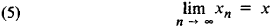then we can speak of continuous transformations. A transformation f is said to be continuous if equation (5) implies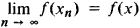The set of all continuous transformations forms a group of continuous transformations. In many cases (but not always) there is a natural way of topologizing a group of continuous transformations, that is, of defining in the group the concept of passage to a limit. Then we speak of the convergence of a sequence of transformations to a transformation. It turns out here that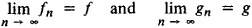implies that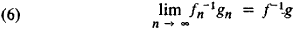Such a group is called a topological transformation group.

Suppose M is a set of points in the plane. A transformation f is said to be a motion of the plane if for every pair of points x and y in M, the distance between x and y is equal to the distance between f (x) and f (y). A transformation of the plane is said to be projective if collinear points are carried into collinear points. A projective transformation that carries parallel lines into parallel lines is called an affine transformation. Here we have the three very simple geometric examples of topological transformation groups, namely, the group of motions, the group of projective transformations, and the group of affine transformations. If we consider those properties of geometric figures in the plane that remain invariant under motions of the plane, then we obtain ordinary elementary geometry. Projective and affine geometries arise in an analogous fashion.

F. Klein advanced a general point of view (the Erlangen program) according to which geometry is a science that studies those properties of figures that remain invariant under a given group of continuous transformations. In this way topological groups entered geometry. Let M be the set of all ordered n-tuples of numbers x1, x2,. . . , xn, which we regard as components of the vector x. We consider the linear transformation f that carries the vector xinto a vector y with components y1, y2, . . . , yn, where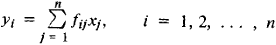The set of all linear transformations constitutes a topological transformation group. We may consider not all linear transformations, but, for example, those that preserve the lengths of vectors, that is, those for which

x12 + x22 + . . . + xn2 ═ y12 + y22 + . . . + yn2

Such transformations form the group of orthogonal linear transformations. Groups of linear transformations are extremely important. In particular, such groups are applied in quantum mechanics.

The modern development of group theory has shown that in studying groups it is worthwhile to ignore the fact that the elements of a group are transformations and to treat a group as a set of elements on which there is defined an operation of multiplication that associates to each pair of elements f, g their product k ═ fg and that satisfies (2), (3), and (4). These conditions are now the group axioms. The element e, previously the identity transformation, is now called the identity element of a group. An inverse element replaces the inverse transformation. The existence of an identity element and the existence of an inverse element are now axioms. If for any two elements f and g, the equality fg ═ gf is valid, then the group is said to be commutative. In order to obtain a topological group, it is necessary to assume that the elements of the group constitute a topological space and that the multiplication operation is continuous, that is, condition (6), now an axiom, holds.

In this way, a new, abstract concept of a continuous, topological group, entered mathematics. Logically, a topological group is a set on which there are defined operations of multiplication and passage to the limit. Since both these operations are very frequently encountered in mathematics, the concept of a topological group is very important and has numerous applications. Lie groups (after S. Lie, the founder of the theory of topological groups) are an important type of topological group. If coordinates can be introduced in a neighborhood of the identity element of a group—that is, if every element f can be specified by numbers f1, f2, . . . , fr, which are the coordinates of f—then for elements close to the identity element, the multiplication rule k ═ fg can be written in coordinate form:

(7) ki ═ ϕi (f1, f2, . . . , fr; g1, g2, . . ., gr

i ═ 1, 2, . . . , r

where ϕi is a continuous function of all its variables. If we further assume that ϕi is three times continuously differentiable, we arrive at the concept of a Lie group. If we assume that the coordinates of the identity element are all equal to zero, that is, if the identity element is taken as the origin, then by expanding the right side of equation (7) in a Taylor series we obtain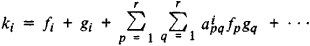The numbers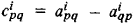are called the structure constants of the Lie group, and the study of the Lie group entirely reduces to the study of these constants.

### REFERENCE

Pontriagin, L. S. Nepreryvnye gruppy, 3rd ed. Moscow, 1973. (Contains a bibliography.)

L. S. PONTRIAGIN